# 10. Consider the situations of a lender of money and a borrower of money. Which of...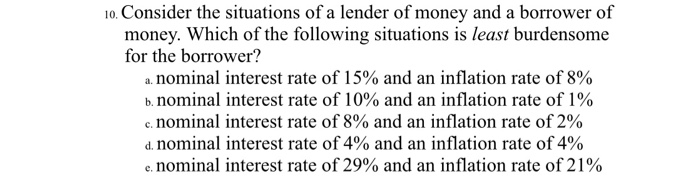10. Consider the situations of a lender of money and a borrower of money. Which of the following situations is least burdensome for the borrower? a. nominal interest rate of 15% and an inflation rate of 8% b. nominal interest rate of 10% and an inflation rate of 1% c. nominal interest rate of 8% and an inflation rate of 2% d. nominal interest rate of 4% and an inflation rate of 4% e. nominal interest rate of 29% and an inflation rate of 21%

SOLUTION :

Borrower pays interest on the money borrowed to the lender. From the point of view of the borrower, lower the inflation adjusted interest rate (real rate) better it is. In that case borrower’s financial cost is lower.

Accordingly , the lowest real rate should be the choice of the borrower .

In this case :

Nominal rate            Inflation rate          Real rate (Nominal- Inflation)

15%                           8%                              7%

10%                           1%                              9%

8%                            2%                              6%

4%                            4%                              0%

29%                          21%                             8%

So,

Nominal rate of 4% and inflation rate of 4% result into the real rate of 0%which is the lowest of all situations and so, this situation is the least burdensome for the borrower. (ANSWER).

Ans is D

Borrower is least burden some when the real interest rate is lowest

and real interest rate=Nominal interest rate-inflation rate

option D has real interest rate=0 because Nominal interest rate=inflation rate

#### Earn Coin

Coins can be redeemed for fabulous gifts.

Similar Homework Help Questions
• ### 4. What is the value of the real interest rate in each of the following situations?...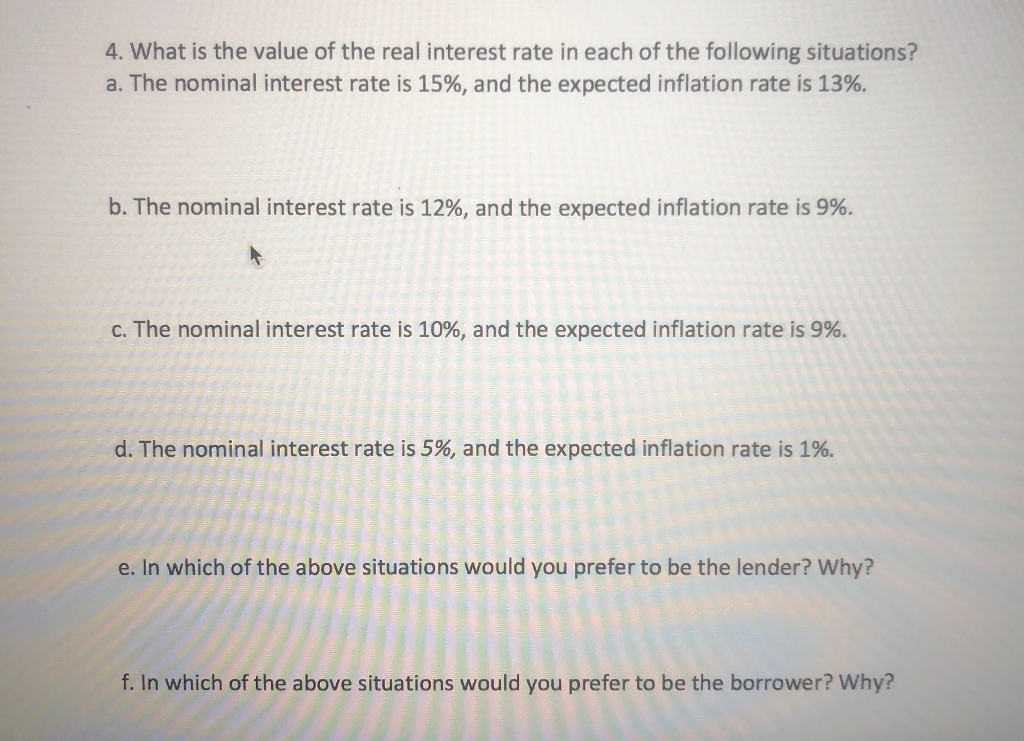4. What is the value of the real interest rate in each of the following situations? a. The nominal interest rate is 15%, and the expected inflation rate is 13%. b. The nominal interest rate is 12%, and the expected inflation rate is 9%. c. The nominal interest rate is 10%, and the expected inflation rate is 9%. d. The nominal interest rate is 5%, and the expected inflation rate is 1%. e. In which of the above situations would...

• ### 9. Suppose that a borrower and a lender agree on the nominal interest rate to be...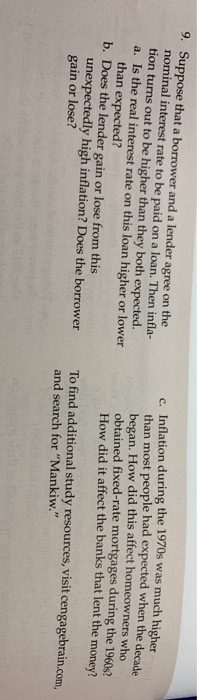9. Suppose that a borrower and a lender agree on the nominal interest rate to be paid on a loan. Then infla- tion turns out to be higher than they both expected. a. Is the real interest rate on this loan higher or lower than expected? b. Does the lender gain or lose from this unexpectedly high inflation? Does the borrower c. Inflation during the 1970s was much higher than most people had expected when the decade began. How did...

• ### A borrower and a lender agree on a mortgage interest rate. If inflation turns out to...

A borrower and a lender agree on a mortgage interest rate. If inflation turns out to be less than expected A. the actual real interest rate will be less than the expected real interest rate. B. the actual nominal interest rate will be higher than expected. C. the actual nominal interest rate will be less than expected. D. the actual real interest rate will exceed the expected real interest rate.

• ### 24. The movement of money from the lender to the borrower and back to savers is...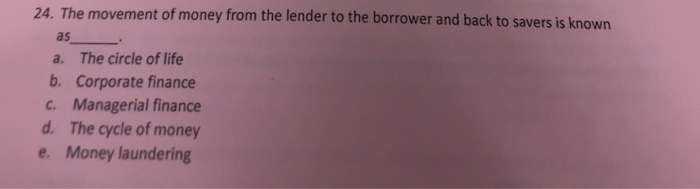24. The movement of money from the lender to the borrower and back to savers is known as a. The circle of life b. Corporate finance c. Managerial finance d. The cycle of money e. Money laundering

• ### answer all of them 29. Which of the following is a real variable? 26. A lender...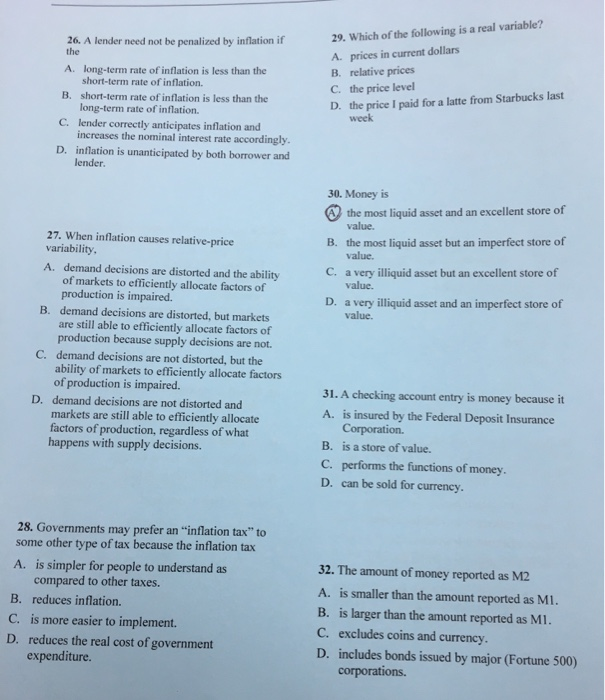answer all of them 29. Which of the following is a real variable? 26. A lender need not be penalized by inflation if the A. prices in current dollars B. relative prices C. the price level A. long-term rate of inflation is less than the short-term rate of inflation. short-term rate of inflation is less than the B. long-term rate of inflation. the price I paid for a latte from Starbucks last week D. C. lender correctly anticipates inflation and...

• ### O Cucome my price cente proportionat cater than the inten nominal income Yes, because real income...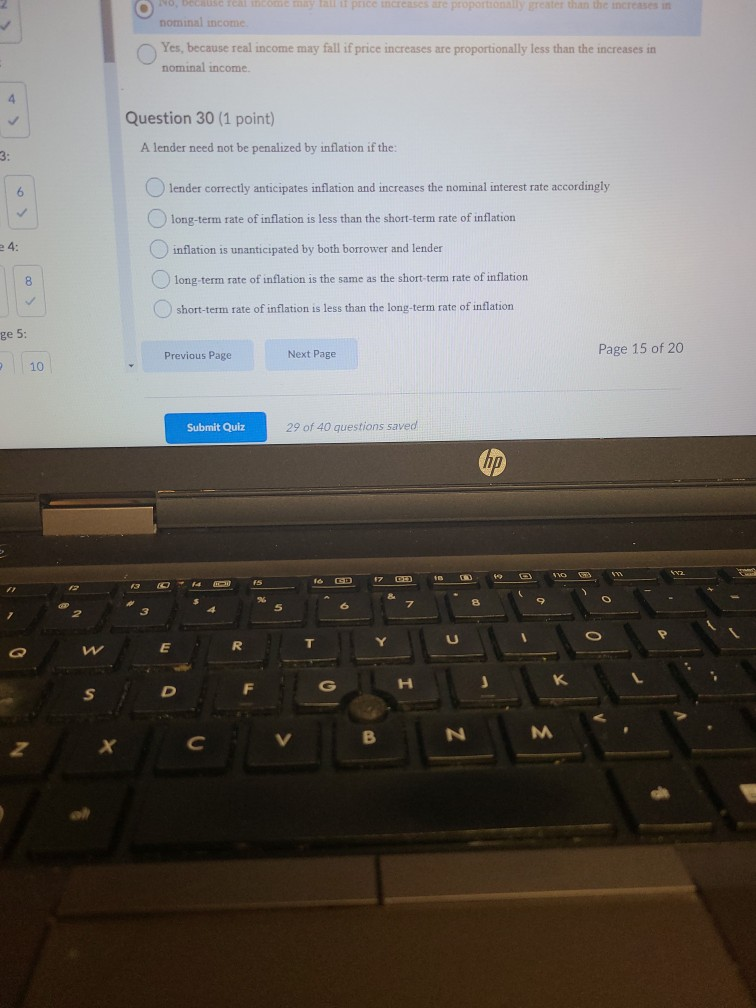O Cucome my price cente proportionat cater than the inten nominal income Yes, because real income may fall if price increases are proportionally less than the increases in nominal income 4 Question 30 (1 point) A lender need not be penalized by inflation if the: 3: 6 lender correctly anticipates inflation and increases the nominal interest rate accordingly ulong-term rate of inflation is less than the short-term rate of inflation 4: inflation is unanticipated by both borrower and lender 8...

• ### Considering the idea of the real rate of interest, ir. In which of the following situations...

Considering the idea of the real rate of interest, ir. In which of the following situations would you prefer to be the lender? a. The interest rate is 9 percent and the expected inflation rate is 7 percent. b. The interest rate is 4 percent and the expected inflation rate is 1 percent. c. The interest rate is 13 percent and the expected inflation rate is 15 percent. d. The interest rate is 25 percent and the expected inflation rate...

• ### 17. Consider two countries: Canada and the United States. The income elasticity of money demand is...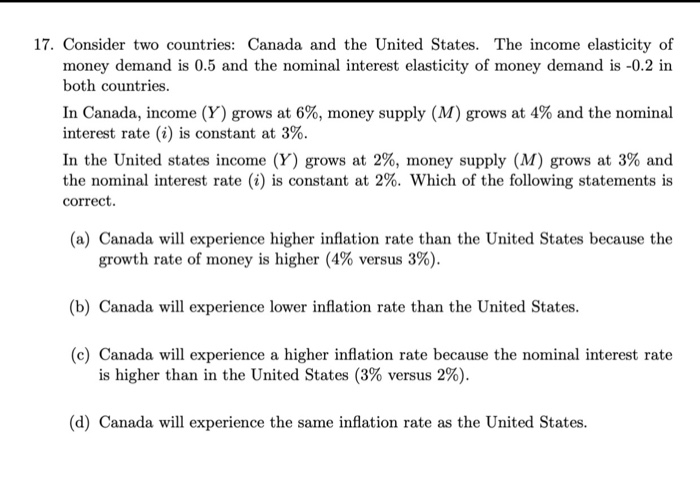17. Consider two countries: Canada and the United States. The income elasticity of money demand is 0.5 and the nominal interest elasticity of money demand is -0.2 in both countries. In Canada, income (Y) grows at 6%, money supply (M) grows at 4% and the nominal interest rate (i) is constant at 3%. In the United states income (Y) grows at 2%, money supply (M) grows at 3% and the nominal interest rate () is constant at 2%. Which of...

• ### Calculate the inflation rate.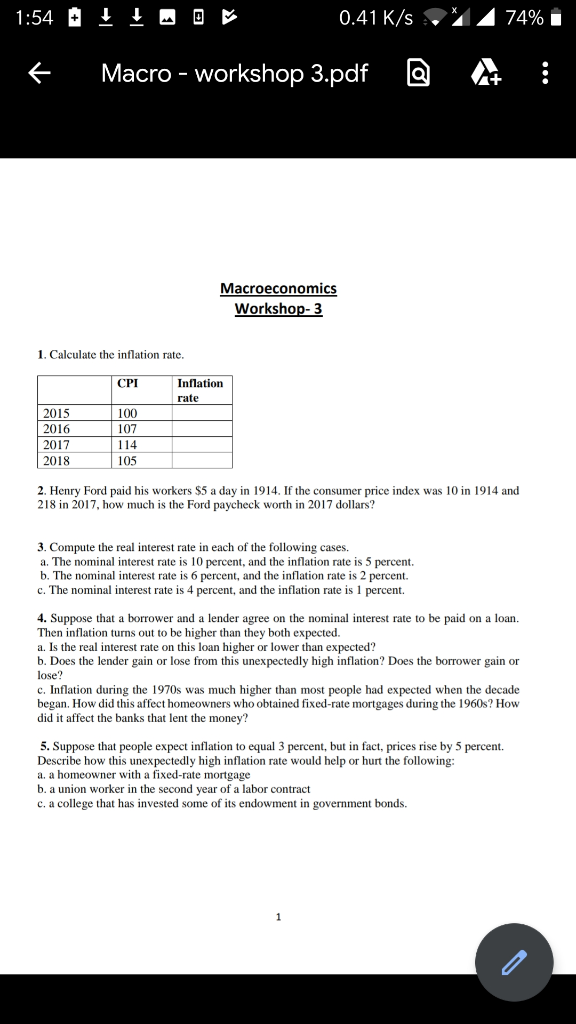1. Calculate the inflation rate. CPIInflation rate 20151002016107201711420181052. Henry Ford paid his workers \$5 a day in 1914. If the consumer price index was 10 in 1914 and 218 in 2017, how much is the Ford paycheck worth in 2017 dollars? 3. Compute the real interest rate in each of the following cases. a. The nominal interest rate is 10 percent, and the inflation rate is 5 percent. b. The nominal interest rate is 6 percent, and the inflation rate is 2 percent....

• ### 7) Which of the following 10-year, \$10,000 face-value securities would you buy based on the yield...

7) Which of the following 10-year, \$10,000 face-value securities would you buy based on the yield to maturity criterion? A) A 5 percent coupon bond selling for \$10,100                       B) A 10 percent coupon bond selling for \$10,000 C) A 15 percent coupon bond selling for \$10,000                     D) A 15 percent coupon bond selling for \$9,900 8) The future value of a security is A) inversely related to the market interest rate.                 B) directly related to the time until...

Free Homework Help App